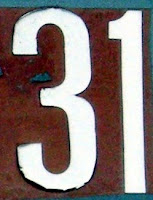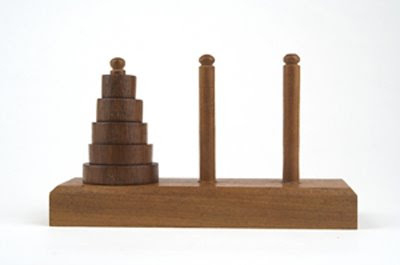## Friday, March 20, 2009

### 31

31 is a prime number. It is also a Mersenne number (of the form 2p - 1, where p = 5).

31 is the sum of the first two primes raised to themselves: 31 = 22 + 33.

31 is the smallest number with exactly two representations as a sum of four squares: 31 = 12 + 12 + 22 + 52 = 22 + 32 + 32 + 32.

There are only
31 numbers that cannot be expressed as the sum of distinct squares.

29 and 31 form a twin prime pair.

31 is 11111 in base 2 (binary).

31 = 50 + 51 + 52, which means it is 111 in base 5.

31 is a number that cannot be written as a sum of three squares.31 is the minimum number of moves required to solve a Tower of Hanoi puzzle containing five discs. The Tower of Hanoi puzzle consists of three rods and a given number of discs that can slide onto any rod. The discs start as an ordered stack on one rod, with the largest at the bottom and the smallest on top. The objective is to move all the discs from one rod to another rod, with the discs again stacked in the correct order, subject to the following rules: only one disc may be moved at a time; each move consists of taking the upper disk from one of the rods and sliding it onto another rod, on top of the other disks that may already be present on that rod; and no disc may be placed atop a smaller disc.Source:
Number Gossip; Cut the Knot